### Home > CALC > Chapter 4 > Lesson 4.1.2 > Problem4-22

4-22.
1. The value of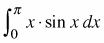is π. Homework Help ✎1. Without a calculator, find: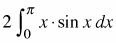and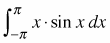.

2. Use a graph to justify your conclusion.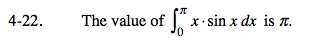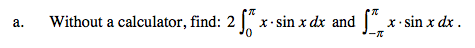Is f(x) = xsin(x) even, odd or neither?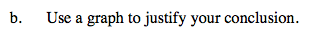f(a) = asin(a)
f(−a) = −asin(−a)
but, since y = sinx is odd,
= −a(−sin(a)) = asin(a)
So f(a) = f(−a)
therefore, y = xsinx is even.

Use the eTool below to examine the graph.
Click the link at right for the full version of the eTool: Calc 4-22 HW eTool